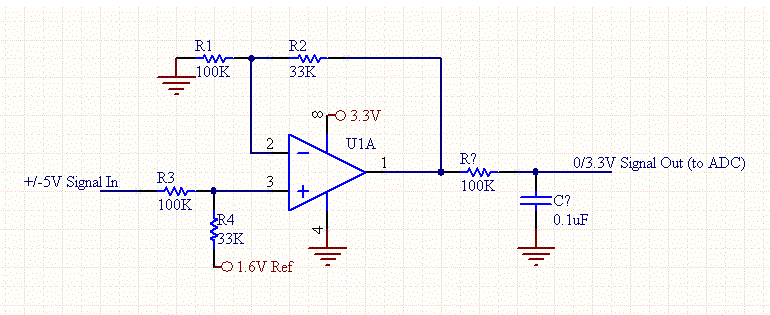# Non-Inverting Op-Amp Level Shifter

A common engineering task is to convert a positive to negative signal into a range suitable for a single supply ADC. This circuit will convert a +/-5V signal into a 0 to 3.3V signal so that it can be sampled by an ADC on a 3.3V microprocessor.Check out our Op-Amp Resistor-Calculator.

Equations:

A= (R4/R1) x (R1+R2)/(R3+R4)

If R1= R3, and R2=R4, then

A= (R4/R1)

We want to convert a 10Vpp signal to a 3.3V signal so the gain should be 1/3. We can choose R4 to be 33K and R1 to be 100K.

Now we need to choose the positive offset such that the signal is centered at 1.6V.

The gain off the offset voltage is:

Aoffset= (R2+R1)/R1 x R3/(R3+R4) = R3/R1.

For the previous resistor values, the gain is 1 since R3=R1, and so we use an offset voltage of 1.6V.

[Plant Database], [Soil Moisture Sensor] [Water Level Sensor] [Soil Moisture Meter]